# How to calculate days until next birthday in Excel?

How would you find out how many days are left till your next birthday, if it is something you are interested in?  Actually, if you are counting down the days till your next birthday with Microsoft Excel, you may utilise the worksheet formulae to make the process much simpler.

Let’s understand step by step with an example.

## Step 1

Make a worksheet where the Headers are displayed below.

Create column headings in a worksheet in a sequential order, as demonstrated in the screenshot below. In addition, enter your date of birth into the cell labelled "DOB " in column B2 of the spreadsheet.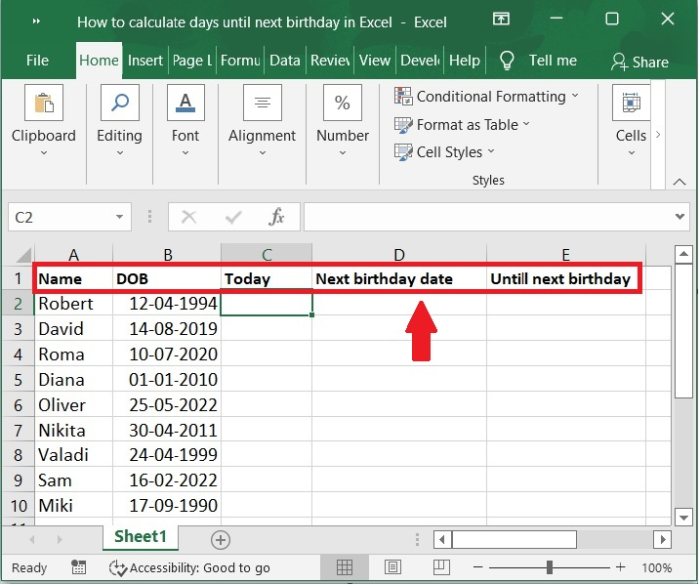## Step 2

Find out today's date. We need to use a formula to figure out what today's date is. Choose cell C2 and then paste the formula that is provided below into it before pressing the Enter key. Please refer to the screenshot below.

=TODAY()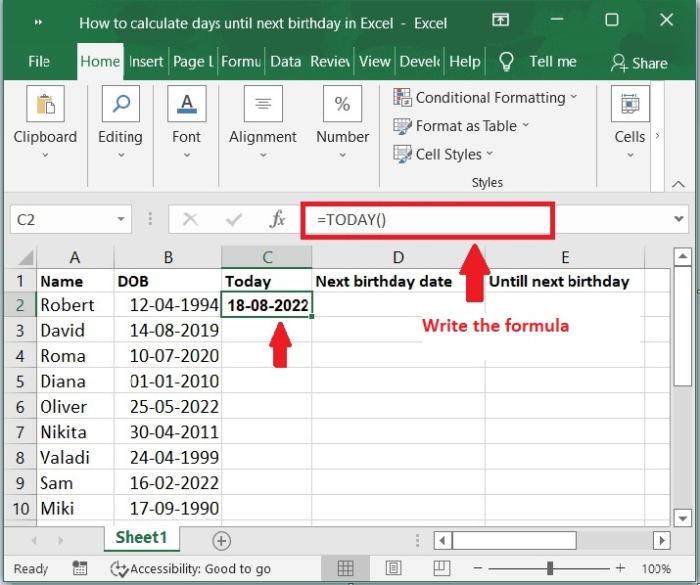## Step 3

After that, the date for today will appear in the second cell of the C column. Now drag to fill handle to the bottom of its range. Please check out the screenshot below.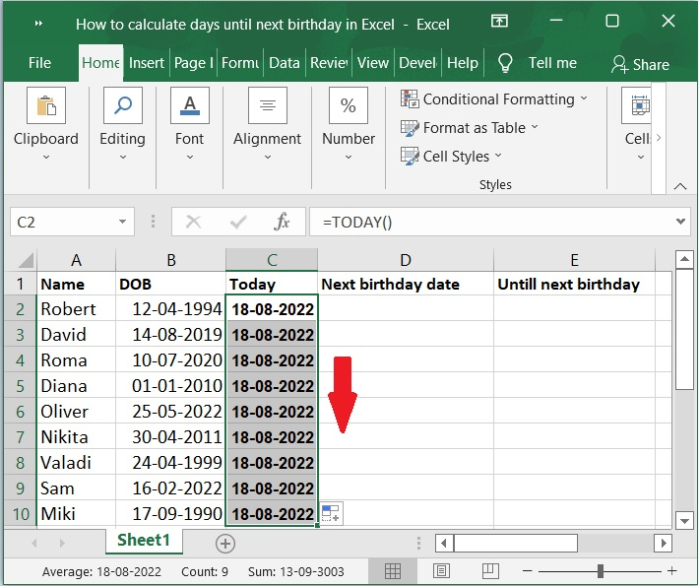### Step 4

Find out when your birthday is next year.

Choose cell D2, paste the formula shown below into it by hand or by copying it, then hit the Enter key. As shown in the below screenshot for the same.

=DATE(YEAR(B2)+DATEDIF(B2,C2,"y")+1,MONTH(B2),DAY(B2))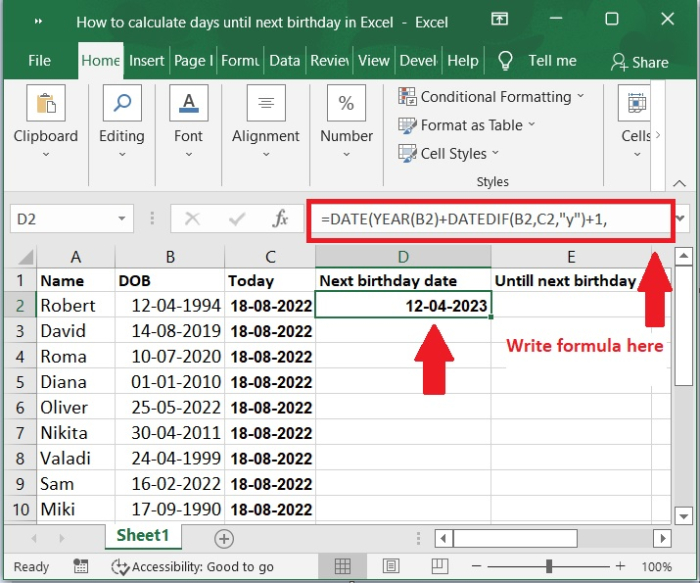### Step 5

Now, drag to fill handle to the bottom of its range. Please check out the screenshot below for the same.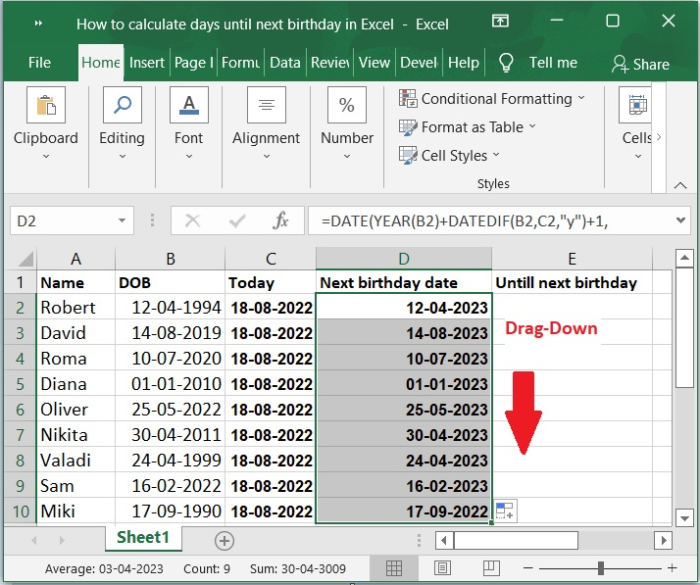## Step 6

Find out how many days are left until your next birthday.

Choose cell E2 and then paste the formula shown below into it before pressing the Enter key. Please check out the below screenshot.

=DATEDIF(C2,D2,"d")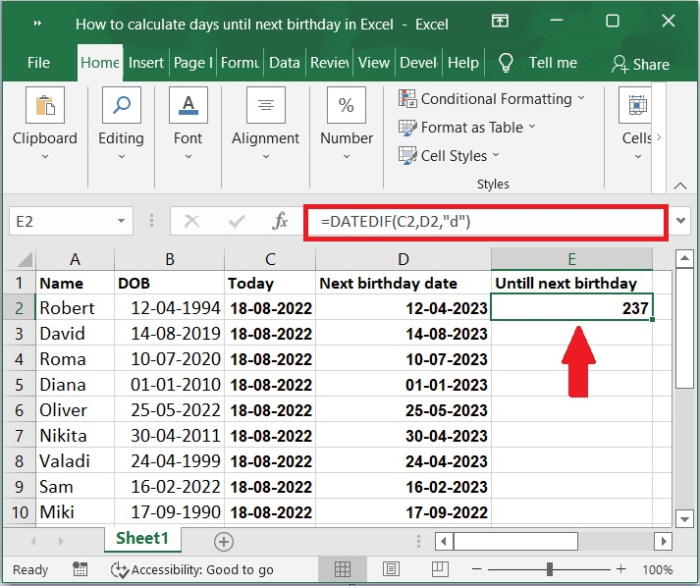## Step 7

The number of remaining days before your next birthday will be displayed in cell E2 and drag to fill handle to the bottom of its range. Please check out the screenshot below for the same.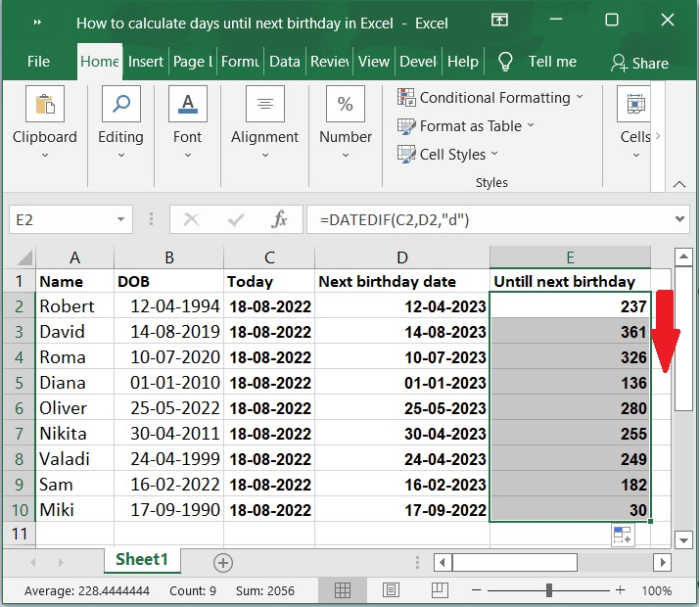Important Note − In addition to the above-mentioned formulas, we also have one that will do you a solid if you insert your birth date into a blank cell (say, B1) and then enter the formula below. To find out how many days are left till your next birthday, type the formula −

"=IF(B1,DATE(YEAR(B1)+DATEDIF(B1+1,TODAY(),"y")+1,MONTH(B1),DAY(B1))-TODAY(),"")" and hit the Enter key.

## Conclusion

We hope this tutorial helped you understand how to calculate days until next birthday using formulas in Excel.

Updated on: 03-Feb-2023

982 Views